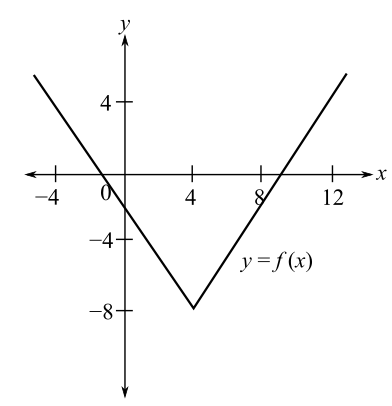Chapter 9.1, Problem 1E### Mathematical Applications for the ...

11th Edition
Ronald J. Harshbarger + 1 other
ISBN: 9781305108042

#### Solutions

Chapter
Section### Mathematical Applications for the ...

11th Edition
Ronald J. Harshbarger + 1 other
ISBN: 9781305108042
Textbook Problem

# In Problems 1 -6, a graph of y   =   f ( x ) is shown and a c-value is given. For each problem, use the graph to find the following, whenever they exist.(a) lim x → c f ( x ) and (b) f ( c ) c = 4(a)

To determine

The value of limxcf(x) by the use of the graph,Explanation

Given Information:

The graph of y=f(x) is

The value of c is 4.

Explanation:

Consider the provided graph,

The value of c is equal to 4.

The limit from the left is represented by limx4f(x) and the limit from the right is represented by limx4+f(x). The limxcf(x) will exist at c=4 when the limit from the left, that is, the values of f(c) but c<4, is equal to the limit from the right, that is, the values of f(c) but c>4

(b)

To determine

The value of f(c) by the use of the graph,### Still sussing out bartleby?

Check out a sample textbook solution.

See a sample solution

#### The Solution to Your Study Problems

Bartleby provides explanations to thousands of textbook problems written by our experts, many with advanced degrees!

Get Started

#### Find more solutions based on key concepts